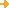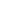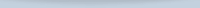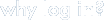search:advanced search
(354 resources)

Resources

 Mathworld, hosted and sponsored by Wolfram Research, Inc., is an online mathematics encyclopedia intended for students, educators, math enthusiasts, and researchers. This amazing resource was compiled over 12 years by... https://mathworld.wolfram.com/ This website demonstrates various math concepts and problems. The topics are organized by topic, such as math for everyone, general math, K-8 math, algebra, plots & geometry, and trigonometry & calculus. Each... http://www.webmath.com/ZweigMedia Finite Mathematics & Applied Calculus Stefan Waner and Steven Costenoble of Hofstra University's Department of Mathematics (mentioned in the February 14, 1997 Scout Report) provide the Finite Mathematics & Applied Calculus Resource Website as a parallel... https://www.zweigmedia.com/index.php?lang=en QuickMath is one of the most useful sites for common but tedious mathematical calculations. It is a free "online calculator that solves equations and does all sorts of algebra and calculus problems." Separated into many... https://quickmath.com/ This is the homepage of an online journal that disseminates "articles that significantly advance the study of categorical algebra or methods, or that make significant new contributions to mathematical science using... http://www.tac.mta.ca/tac/

Switch to browsing by LCC (More Detailed Classifications)
Switch to browsing by Key Conceptforget your password?Save, organize, and share resources that you find.

Subscribe to bulletins

It's easy, fast, and FREE!get your own loginAMSER =
FREE ONLINE
RESOURCES
for the
CLASSROOM